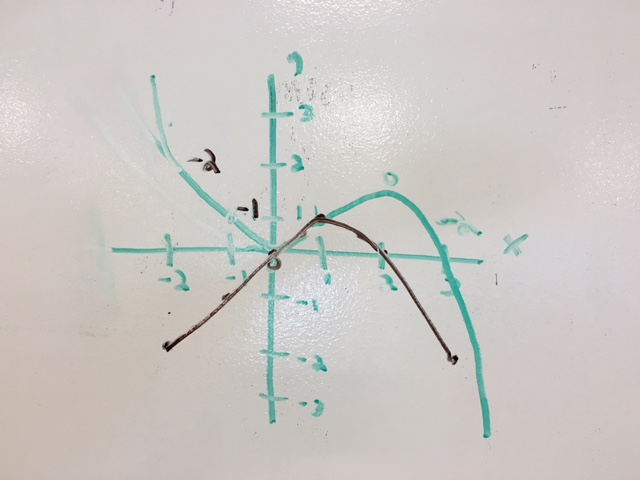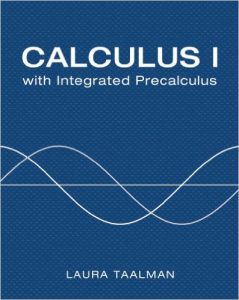# Mon 8/29 – Hello & Slope Functions

First day of class! Today we’ll get to know each other, make sure you’re all in the right class, discuss the goals of the course, and even have a chance to do a little math. If we have time at the end then we’ll take a visit to the Math and Science Learning Center.

### // Before class

• Make sure that MATH 231 is the correct class for you. You should be in MATH 231 if your Calculus Placement Score is between 18 and 29 and you are a potential Math or Science major but not a Biology major. Biology majors should take MATH 233E instead of MATH 231.
• Make sure that you are registered for the correct section of MATH 231: Section 03 at 9:05-9:55 MWF, or Section 04 at 10:10-11:00 MWF.
• If you are on the wait list for Section 03 or Section 04, then you are welcome and encouraged to attend class while you wait for a slot to open.

### // From the board

• Here is one group’s solution to the slope function sketching problem from the end of class. The green graph is the original, and the black graph is its associated slope function. Notice that approximate slopes are labeled along the green graph; these slopes are then used to plot the heights of the black graph. In Chapter 2 we’ll revisit this type of problem when we study derivatives.### // After class

• Get a spiral or otherwise bound paper Notebook for this class.• If you haven’t already, get the textbook: Calculus 1 with Integrated Precalculus by Taalman. You can get it used or new or maybe even rented, as long as it looks like the picture on the right.
• From the textbook, read the following three review sections:
• Section 0.1: Numbers and Sets
• Section 0.2: Equations
• Section 0.3: Inequalities
• Take notes from these three sections in your Notebook. You will be able to use these notes in the Daily Quiz at the start of Wednesday’s class.
• Look over the homework problems for these three sections of the textbook, and get a sense for which problems you think you might want to ask about in class.
• If you’re in MATH 199, then log into and start MyMathTest right away; get qualified to attempt at least one Gateway Test by Friday’s proctored session.
• Take a 5-minute Daily Quiz on Sections 0.1, 0.2, and 0.3 at the start of class,
• List specific questions about the reading and examples from these three sections, and
• Suggest types of homework exercises for class group work.

# Before the first day of class

Hello and welcome to Math 231. My name is Laura and I am excited to meet you on the first day of class.  Here are some things that you might want to consider before that first day.

### // What to expect from MATH 231 in Sections 03 and 04

All sections of MATH 231 use the same textbook, but each MATH 231 instructor implements their own style and philosophy of teaching. Here’s what it will be like in my sections of MATH 231 this semester:

• Class will meet in EPIC classroom 4043 in the Student Success Center, which is a room outfitted with group-work tables and whiteboard-painted walls.
• Class meetings will consist primarily of active group work and presentations, rather than a lecture format.
• You will be expected to read each section and begin attempting examples and homework exercises before each class day.

If the type of class described above does not appeal to you, then you might prefer another section. On the other hand, if it just sounds a little unfamiliar and scary, then you’ll be fine. It will be a welcoming and friendly class atmosphere in which exploration, mistakes, and even failures are embraced on a daily basis as you all learn the material.

### // If you’re on the Wait List for Section 03 or 04

If you are on the Wait List to get into Section 03 or 04 of MATH 231 and are still hoping to get into the class, then I encourage you to attend class each day, if posssible. That way you won’t start at a disadvantage if you do get into the section.

### // Should you be in MATH 231?

MATH 231 is for Math and Science majors who need to bolster their precalculus and algebra knowledge.  As the course catalog says:

MATH 231 and MATH 232 form a sequence that combines first-semester calculus with algebra and trigonometry. The sequence is designed for students whose pre-calculus skills are not strong enough for MATH 235.

Note that the determining factor for inclusion in MATH 231 rather than MATH 235 is proficiency in Precalculus and Algebra topics, not in Calculus. About half of the students who take MATH 231 have already taken a Calculus course in high school.

The Calculus Placement Test consists of 45 questions from Precalculus and Algebra and is a test of readiness for calculus, not a test of calculus topics. MATH 231 students should have Calculus Placement Test (CPT) scores between 18 and 29 (inclusive).

• If you scored less than an 18 on the CPT, then you should take MATH 155 or MATH 156 before enrolling in MATH 231.
• If you scored between 18 and 22 on the CPT, then you are required to take Math 199 concurrently with MATH 231.
• If you scored 30 or higher on the CPT, then you should consider taking MATH 235.

MATH 231-232 is a Calculus sequence that is equivalent to MATH 235 for all prerequisites and requirements. That means that the material in MATH 231-232 is at a level equally as rigorous and challenging as the material in MATH 235, and suitable for students majoring in Math or Science. MATH 231 is for potential Math and Science majors except for Biology majors, who need to take a different calculus sequence.

• If you are a Biology major who scored less than 30 on the CPT, then you should take MATH 233-234 instead of MATH 231-232.
• If you are not a Math or Science major, then you are still welcome to take MATH 231; however, you may have other options for fulfilling your mathematics requirements at JMU.

### // If you’re also in MATH 199

Historically, students who score less than 23 on the Calculus Placement Test (CPT) have a difficult time succeeding in MATH 231 without additional help in Algebra. To solve this problem we created MATH 199, which is a self-paced required co-requisite course for MATH 231 students who score between 18 and 22 on the CPT.

Grades in MATH 199 are determined solely by the number of weeks that it takes to successfully pass five Gateway Tests. Once you pass all five Gateway Tests you will be done with MATH 199. If you are enrolled in MATH 199 then you should make it a priority to work on the online assignments and practice tests in order to pass the Gateway Tests as soon as possible.

Passing MATH 199 quickly will not only get you a better grade in MATH 199, it will also help you succeed in MATH 231. Please reach out to me if you have any questions regarding MATH 199 and how you can best succeed in that course.

1 4 5 6